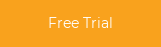# Use MATLAB to Leverage Your Live IoT DataIf you have ever done any data analysis from a sensor or other type of data source, you have most likely followed a process where you collect the data, you convert the data and then use MATLAB to process and analyze the data.  Using  MATLAB to analyze the data is a very well known tool to accomplish that task.  Collecting and converting the data, so that it is usable in  MATLAB, can take an enormous amount time.  Thanks to an integration that was completed by MathWorks, it is now possible to easily connect  MATLAB up with live data that is being published and subscribed to on DDS.  With  MATLAB being one of the top tools used to analyze data and DDS quickly becoming the data communications middleware of IIoT applications, this integration will enable some very rapid prototyping and test analysis for developers.  This blog post will walk through a few examples of how to publish DDS data and also how to subscribe to DDS data using  MATLAB.

## Getting Started

To get started, you will need to make sure that both  MATLAB and RTI Connext DDS are installed on your computer.  For this set of examples, the following versions were used:

Once you have those installed, just follow the video at this link to complete and verify the installation:  Installation Video

## Initialization

Once you have everything installed and verified, then there are just a few steps to get DDS setup appropriately within  MATLAB.

•  Import the datatype(s) that will be used in your project.
•  Create a DDS Domain Participant
•  Create a DDS DataWriter

Importing a datatype in  MATLAB is simple.  In DDS, datatypes are specified using IDL files.  The  MATLAB import statement can read an IDL file directly and will create the ".m" files required to work with that datatype within the  MATLAB interpreter.  The following  MATLAB call will import a datatype called "ShapeType" from the ShapeType.idl file located in the current working directory:

`>> DDS.import('ShapeType.idl','matlab','f')`

Now that datatype is available to use when creating your DataReaders and DataWriters of topics in DDS.  Also note, that once the import has been done, this step no longer has to be run in the future.  The type will be available in  MATLAB going forward.  The next thing to do to get DDS discovery going is to create a DDS Domain Participant.  That can be accomplished in this call:

`>> dp = DDS.DomainParticipant;`

Using this DomainParticipant (dp) object, you can then create both DataWriter and DataReader objects.  The following two commands will add a datawriter object and datareader object to the dp specifying its type to be the newly created "ShapeType" and their topics to be "Triangle" and "Square" respectively.

```>> dp.addWriter('ShapeType','Triangle')

## Subscribing to Data in Shapes Demo

The ShapeType is used so that it will communicate with the standard RTI Shapes Demonstration application (Shapes) that is provided by RTI.  Shapes enables the creation of both DataWriters and DataReaders of "Square", "Circle" and "Triangle" topics that are in turn based on the "ShapeType" datatype.  For more information on how to use the Shapes application, click here to view our video tutorial.

In Shapes, the next step is to create a subscriber of Triangle. In the next screen just leave all the other QoS options as default.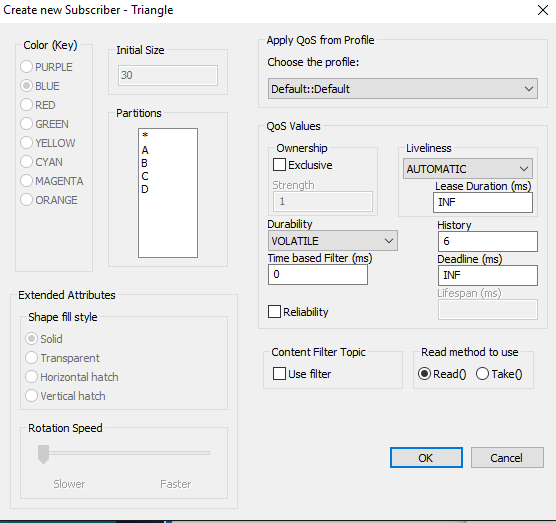## Publishing Data in  MATLAB

Now that we have the DataWriter setup in  MATLAB to send out ShapeType on the Triangle topic, and also we have the Shapes Demo setup to receive the publication, lets exercise the writer.  The following commands will populate the fields of the ShapeType and then publish out the data on the Triangle Topic:

```%% create an instance of ShapeType
myData = ShapeType;
myData.x = int32(75);
myData.y = int32(100);
myData.shapesize = int32(50);
myData.color = 'GREEN';

%% write data to DDS
dp.write(myData);```

The result on the Triangle Topic within the Shapes Demo will be a single Green Triangle shown here: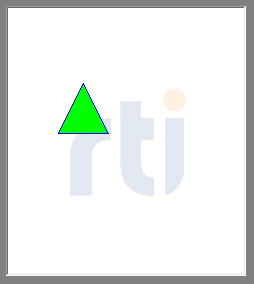Some more interesting use cases of publishing Triangle within  MATLAB are:

```%% Publish out Green Triangles in a line at 1 Hz
for i=1:10
myData.x = int32(20 + 10*i);
myData.y = int32(40 + 10*i);
dp.write(myData);
pause(1);
end

%%  Publish out Green Triangles in a Circle pattern at 20Hz
for i=1:1000
angle = 10*pi * (i/200);
myData.x = int32(100 + (50 * cos(angle)));
myData.y = int32(100 + (50 * sin(angle)));
myData.shapesize = int32(40);
myData.color = 'GREEN';
dp.write(myData);
pause(0.05);
end```

The resulting output on the Shapes Demo are respectively:## Publishing Data in Shapes Demo

In the Shapes demonstration, create a publisher of Square.  In the next screen just pick a color and leave all the other QoS options as default.  The following screenshot shows the Square Publish screen.  For my demonstration, I have chosen an Orange Square.  This will publish the X,Y Position on the screen every 30 msec.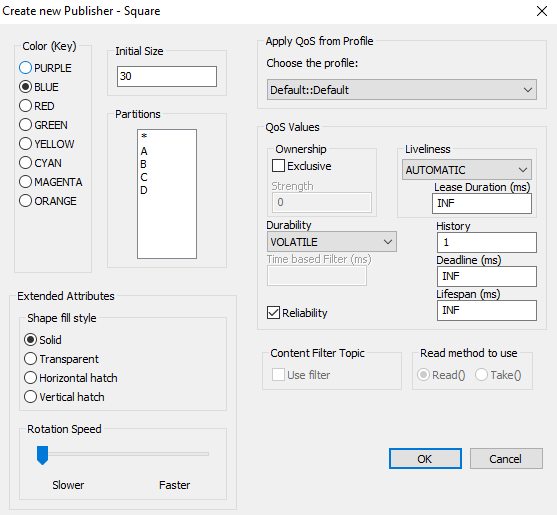## Subscribing to Data in  MATLAB

If you remember from before we added a Square Topic DataReader to the Domain Participant in  MATLAB.  We will use this DataReader to subscribe to data that we are now publishing from the Shapes Demonstration.  The following commands in  MATLAB will read 10 samples at 1 Hz.

```%% read data
for i=1:10
pause(1);
end```

The resulting output in  MATLAB will be 10 reports of the following: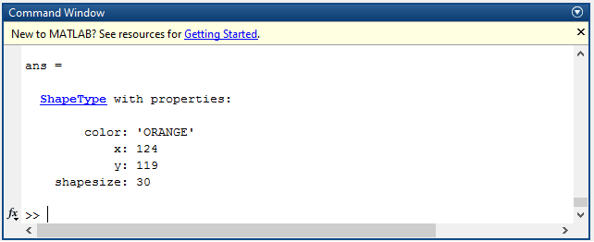## Something More Interesting

Now that we have both directions going, lets do something that is more creative with the data.  First we will read in the Square data and modify it to switch the X and Y coordinates and then republish it out on to a RED Triangle.  Second, we will take the resulting Position data and plot it directly within  MATLAB.  These are the commands to use in  MATLAB to accomplish that.

```%% allocate an array of 100 elements
xArray = zeros(1,100);

%%  run a loop to collect data and store it into the array
%%  also switch up the X and Y coordinates and then republish onto
%%  the Triangle topic
for i=1:100
if ~isempty(myData)
x = myData(1).x;
y = myData(1).y;
xArray(i) = x;
yArray(i) = y;
myData(1).y = x;
myData(1).x = y;
myData(1).color = 'RED';
dp.write(myData(1));
end
pause(0.05)
end

%%  Plot the X Position Data
t = 1:100;
plot(t,xArray);
legend('xPos');
xlabel('Time'), ylabel('Position');
title('X Postions');```

The resulting output in Shapes Demo will be a Red Triangle moving the opposite of the Orange Square and also a Plot will be generated within  MATLAB showing the X Position data: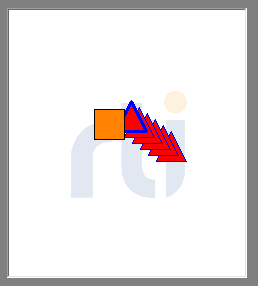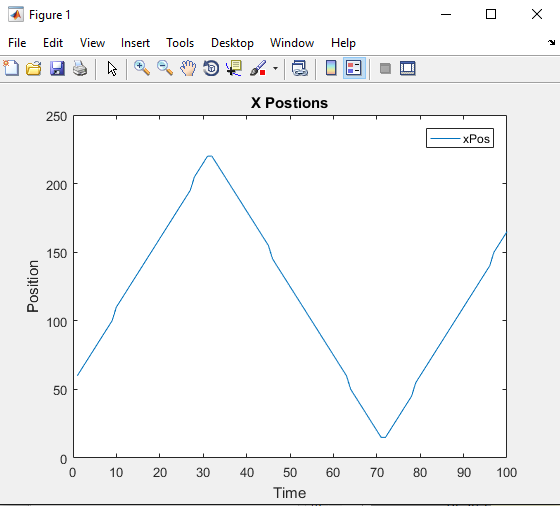As you can see, the integration of DDS with  MATLAB is very simple to use and makes it very easy to collect data, inject data and analyze data.  For this demonstration, we used the simple Shapes Application, but the data used can just as easily be your own application data.  If you would like to find out more about the  MATLAB Integration with RTI Connext DDS, please visit this site on MathWorks site:  MATLAB DDS Integration. If you'd like to learn more about using Connext DDS, click here to gain access to our developer resources.

## Getting Started with Connext

Connext® is the world's leading implementation of the Data Distribution Service (DDS) standard for Real-Time Systems. Try a fully-functional version of Connext for 30 days.